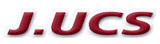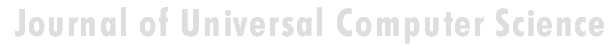Search
 Submission Procedure share: |available in:   PDF (262 kB) PS (194 kB)

get:
 Similar Docs BibTeX Write a comment
get:

DOI:   10.3217/jucs-016-18-2569

### Isometries and Computability Structures

Zvonko Iljazović (University of Zagreb, Croatia)

Abstract: We investigate the relationship between computable metric spaces (Χ, d, α) and (Χ, d, β), where (Χ, d) is a given metric space. In the case of Euclidean space, α and β are equivalent up to isometry, which does not hold in general. We introduce the notion of effectively dispersed metric space and we use it in the proof of the following result: if (Χ, d, α) is effectively totally bounded, then (Χ, d, β) is also effectively totally bounded. This means that the property that a computable metric space is effectively totally bounded (and in particular effectively compact) depends only on the underlying metric space. In the final section of this paper we examine compact metric spaces (Χ, d) such that there are only finitely many isometries Χ → Χ. We prove that in this case a stronger result holds than the previous one: if (Χ, d, α) is effectively totally bounded, then α and β are equivalent. Hence if (Χ, d, α) is effectively totally bounded, then (Χ, d) has a unique computability structure.

Keywords: computability structure, computable metric space, effective compactness, effective dispersion, effective total boundedness, isometry

Categories: F.0, F.1, G.0